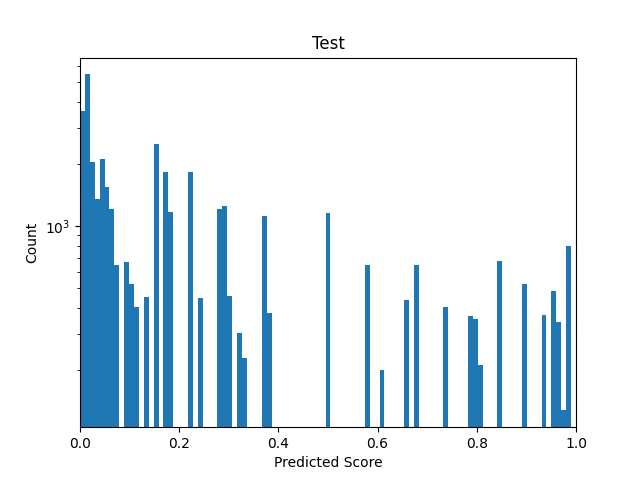# Balance dataset with continous values for student/teacher

Hello,

I want to train a student network and therefore collected all the probability outputs of my teacher (~ 41.000) to create my dataset for the student. The distribution (Binned in 100 bins) looks like this (I already ploted the weights for the WeightedRandomSampler as curve):The weights are obtained by:

``````batch_size = 100
weights = 1. / dataset.targets.bincount().double()
sampler = torch.utils.data.sampler.WeightedRandomSampler(weights=weights, num_samples=len(dataset), replacement=True)

kwargs = {'num_workers': 1, 'pin_memory': True} if device == 'cuda' else {}